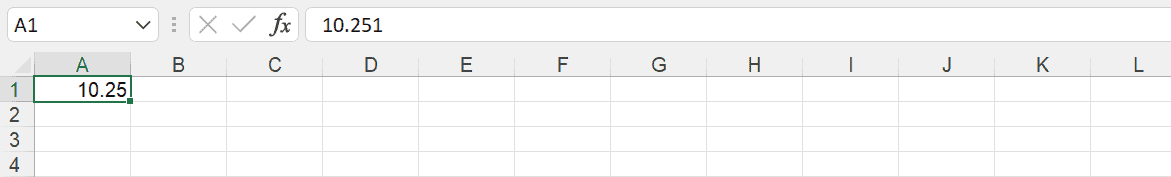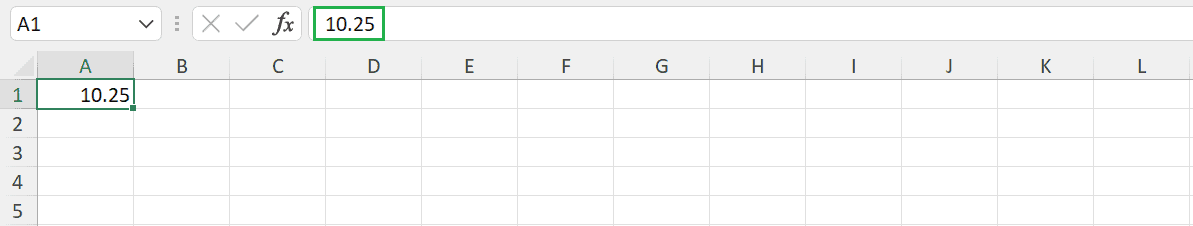### Get started with Spring and Spring Boot, through the Learn Spring course:

>> CHECK OUT THE COURSE

## 1. Overview

In this quick tutorial, we’ll demonstrate how to format numeric cells in Excel using Apache POI.

## 2. Apache POI

Apache POI is an open-source pure Java project. It provides libraries for reading and writing files in Microsoft Office formats, such as Word, PowerPoint, and Excel.

While working with the newer .xlsx file format, we would use the XSSFWorkbook class, and for the .xls format, we use the HSSFWorkbook class.

## 3. Numeric Format

The setCellValue method of Apache POI accepts only double as an input or an input that may be implicitly cast to double and returns a double as a numeric value. The setCellStyle method is used to add the required style. A # character in Excel Number formatting means placing a digit here if required, whereas a character 0 implies always placing a digit here, even if it’s an unnecessary 0.

### 3.1. Format for Display Value Only

Let’s format a double value using patterns like 0.00 or #.##. First, we’ll create a simple utility method for formatting the cell value:

``````public static void applyNumericFormat(Workbook outWorkbook, Row row, Cell cell, Double value, String styleFormat) {
CellStyle style = outWorkbook.createCellStyle();
DataFormat format = outWorkbook.createDataFormat();
style.setDataFormat(format.getFormat(styleFormat));
cell.setCellValue(value);
cell.setCellStyle(style);
}``````

Let’s validate a simple code to validate the mentioned approach:

``````File file = new File("number_test.xlsx");
try (Workbook outWorkbook = new XSSFWorkbook()) {
Sheet sheet = outWorkbook.createSheet("Numeric Sheet");
Row row = sheet.createRow(0);
Cell cell = row.createCell(0);
ExcelNumericFormat.applyNumericFormat(outWorkbook, row, cell, 10.251, "0.00");
FileOutputStream fileOut = new FileOutputStream(file);
outWorkbook.write(fileOut);
fileOut.close();
}``````Note: The display value is the formatted value, but the actual value remains the same. If we try to access the same cell, we will still get 10.251.

Let’s validate the actual value:

``````try (Workbook inWorkbook = new XSSFWorkbook("number_test.xlsx")) {
Sheet sheet = inWorkbook.cloneSheet(0);
Row row = sheet.getRow(0);
Assertions.assertEquals(10.251, row.getCell(0).getNumericCellValue());
}``````

### 3.2. Format for Both Actual and Display Value

Let’s format display and actual values using the patterns:

``````File file = new File("number_test.xlsx");
try (Workbook outWorkbook = new HSSFWorkbook()) {
Sheet sheet = outWorkbook.createSheet("Numeric Sheet");
Row row = sheet.createRow(0);
Cell cell = row.createCell(0);
DecimalFormat df = new DecimalFormat("#,###.##");
ExcelNumericFormat.applyNumericFormat(outWorkbook, row, cell, Double.valueOf(df.format(10.251)), "#,###.##");
FileOutputStream fileOut = new FileOutputStream(file);
outWorkbook.write(fileOut);
fileOut.close();
}``````

This will add the numeric cell in the spreadsheet and display the formatted value, and also, it changes the actual value:Let’s assert the actual value in the above case:

``````try (Workbook inWorkbook = new XSSFWorkbook("number_test.xlsx")) {
Sheet sheet = inWorkbook.cloneSheet(0);
Row row = sheet.getRow(0);
Assertions.assertEquals(10.25, row.getCell(0).getNumericCellValue());
}``````

## 4. Conclusion

In this article, we’ve demonstrated how to display formatted values with or without changing the actual values of numeric cells in an Excel sheet.

All the code samples used in this tutorial are available over on GitHub.

### Get started with Spring and Spring Boot, through the Learn Spring course:

>> CHECK OUT THE COURSE